# Using the api¶

## Basic usage¶

### Simple overlapping winding¶

In the theory section the following simple winding was shown.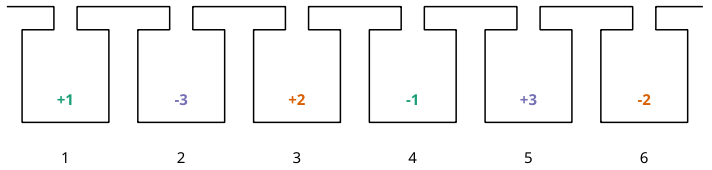Overlapping winding with 6 slots and 2 poles and 3 phases

Let’s have a look how we can model this with swat-em. First of all we need to import swat-em. The relevant part is the datamodel() object. It includes all data and methods for the winding:

```from swat_em import datamodel
```

The model has an built-in winding generator for almost every winding for rotating field motors such as permanent-magnet, synchronous or induction machines:

```>>> wdg = datamodel()      # generate a datamodel for the winding
>>> Q = 6                   # number of slots
>>> P = 2                   # number of pole pairs
>>> wdg.genwdg(Q = Q, P = P, m = 3, layers = 1)
>>> print(wdg)             # print infos for the winding
WINDING DATAMODEL
=================

Title: Untitled
Number of slots:  6
Number of poles:  2
Number of phases: 3
Number of layers: 1
Coil span       : 3
Number of slots per pole per phase: 1
Fundamental winding factor: 1.0, 1.0, 1.0
```

### Simple tooth-coil winding¶

In the same way we can create a tooth-coil winding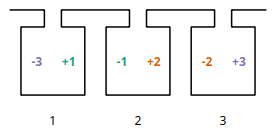Winding layout with 3 slots, 2 poles and 3 phases

We can set the Coil span explicite with the keyword ‘coil_span’. Compared to the overlapping winding we need only 3 slots for the two poles. To get a coil around every tooth, we need two winding layers:

```>>> wdg = datamodel()      # generate a datamodel for the winding
>>> Q = 3                  # number of slots
>>> P = 2                  # number of pole pairs
>>> cs = 1                 # coil span for the coil in slots

>>> # generate winding automatically
>>> wdg.genwdg(Q = Q, P = P, m = 3, layers = 2, coil_span = cs)
>>> print(wdg)             # print infos for the winding
WINDING DATAMODEL
=================

Title: Untitled
Number of slots:  3
Number of poles:  2
Number of phases: 3
Number of layers: 1
Coil span       : 1
Number of slots per pole per phase: 1/2
Fundamental winding factor: 0.866, 0.866, 0.866
```

### A more complex winding¶

A more complex winding (overlapping full pitch winding with coil shortening)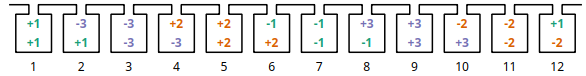Winding layout with 12 slots, 2 poles and 3 phases

```>>> wdg = datamodel()
>>> Q = 12
>>> P = 2
>>> cs = 5     # without shortening coil span would be 6 for this winding
>>> wdg.genwdg(Q = Q, P = P, m = 3, layers = 2, coil_span = cs)
>>> print(wdg)
WINDING DATAMODEL
=================

Title: Untitled
Number of slots:  12
Number of poles:  2
Number of phases: 3
Number of layers: 2
Coil span       : 5
Number of slots per pole per phase: 2
Fundamental winding factor: 0.933, 0.933, 0.933
```

## Results¶

### Getting Results¶

After generating a winding, swat-em analyze it and provides the results:

```>>> wdg = datamodel()
>>> wdg.genwdg(Q = 12, P = 2, m = 3, layers = 1)
>>> print('fundamental winding factor: ', wdg.get_fundamental_windingfactor())
fundamental winding factor:  [0.9659258262890683, 0.9659258262890683, 0.9659258262890684]
>>> print('coil span: ', wdg.get_coilspan())
coil span:  6
```

Get the generated winding layout: For each phase there is a list of the 1st and the 2nd layer. In this example there is only 1 layer, so the second list is empty. An entry of the lists define the slot number in which is a coil-side of the phase is located. A negative number means, that the winding direction is reversed in the slot.

```>>> print('winding layout:', wdg.get_phases())
winding layout: [[[1, 2, -7, -8], []], [[5, 6, -11, -12], []], [[-3, -4, 9, 10], []]]
```

The winding factor depends on the harmonic number. There are two possible interpretations for the harmonic number: The ‘electrical’ harmonic numbers the ‘mechanical’ ordinal numbers multiplyed with number of pole pairs ‘p’. Use the ‘mechanical’ winding factor if you want du determine the possible number of poles your winding can drive and use the electrical winding factor if you know your number of pole pairs and if you want to analyze the harmonic content of the winding for example. Attention: The winding factor is calculated for each phase seperately.

```>>> nu, kw = wdg.get_windingfactor_el()
>>> for k in range(len(nu)):
>>>     print(nu[k], kw[k])
1 [0.96592583 0.96592583 0.96592583]
3 [-0.70710678 -0.70710678 -0.70710678]
5 [-0.25881905 -0.25881905 -0.25881905]
7 [0.25881905 0.25881905 0.25881905]
9 [-0.70710678 -0.70710678 -0.70710678]
...
```

The datamodel() object stores the data in dictionaries. The user have direct access:

```>>> print('Data for the machine: ', wdg.machinedata.keys())
Data for the machine:  dict_keys(['Q', 'p', 'm', 'phases', 'cs', 'turns', 'phasenames'])
>>> # ... and all results:
>>> print('Data for the machine: ', wdg.results.keys())
Data for the machine:  dict_keys(['q', 'nu_el', 'Ei_el', 'kw_el', 'phaseangle_el', 'nu_mech', 'Ei_mech', 'kw_mech', 'phaseangle_mech', 'valid', 'error', 't', 'wdg_is_symmetric', 'wdg_periodic', 'MMK', 'basic_char'])
```

For getting the results the get_* methods can be used:

```>>> print('Is winding symmetric:', wdg.get_is_symmetric())
Is winding symmetric: True
>>> print('Fundamental winding factor:', wdg.get_fundamental_windingfactor())
Fundamental winding factor: [0.9659258262890683, 0.9659258262890683, 0.9659258262890684]
>>> print('Number of turns in series:', wdg.get_num_series_turns())
Number of turns in series: 2.0
Excited radial force modes: [2, 4, 6]
>>> print('Periodictiy:', wdg.get_periodicity_t())
Periodictiy: 1
>>> print('Possible parallel connections:', wdg.get_parallel_connections())
Possible parallel connections: [1, 2]
```

## Plotting¶

### Winding layout¶

SWAT-EM provides some possibilities for graphical representations. After creating a winding one would like to have a look on the layout, for example. This plot includes all coil sides of all phases in the slots:

```>>> wdg = datamodel()
>>> wdg.genwdg(Q = 12, P = 2, m = 3, layers = 1)
>>> wdg.plot_layout('plot_layout.png')
```

### Voltage phasors of the star of slot¶

SWAT-EM calculates the winding factor by the slot voltage phasors. The following is the corresponding visualization.

```>>> wdg.plot_star('plot_star.png')
```

### Winding factor¶

For the winding factor one have to decide between the mechanical or the electrical winding factor. Attention: For a 2-pole machine the electrical and mechanical winding factor is equal.

```>>> wdg.plot_windingfactor('plot_wf.png', mechanical = False)
```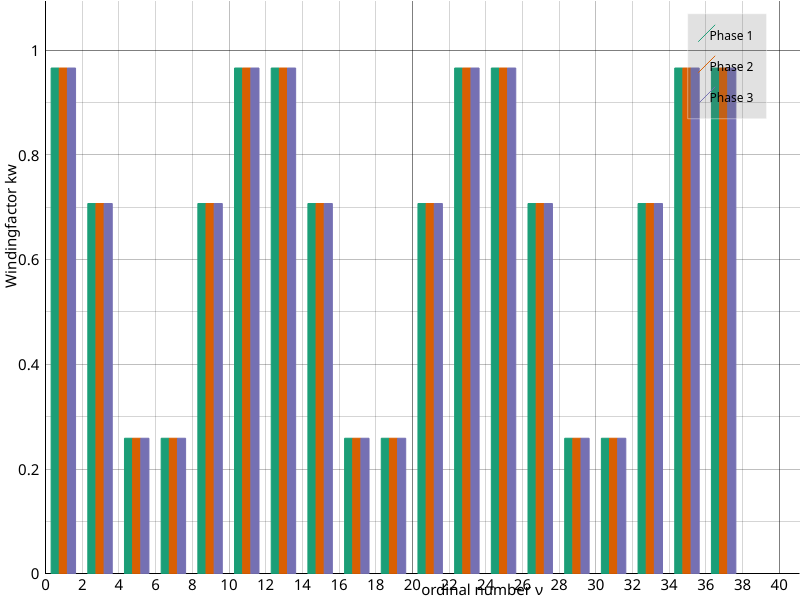Plot of the electrical winding factor

### Magnetomotive force¶

The winding generates a current linkage in the slots. The integral of it leads to a magnetic field in the airgap, which is called the ‘Magnetomotive force (MMF)’. It’s a good indicator for the harmonic content of the winding. Also the resultion of the image can be definded:

```>>> wdg.plot_MMK('plot_MMK.png', res = [800, 600], phase = 0)
```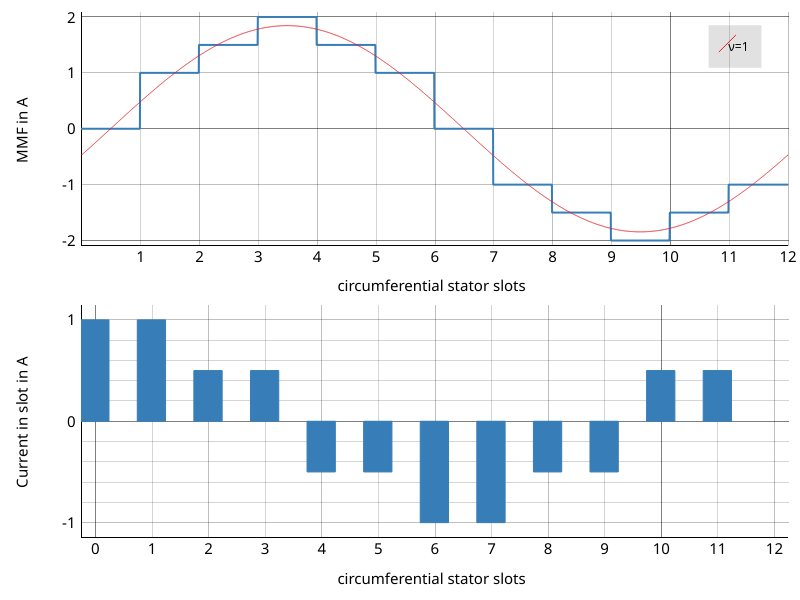Plot of the current linkage in the slots and the resulting Magnetomotove force

It also could be usefull to plot at different phase angles

```>>> wdg.plot_MMK('plot_MMK_20deg.png', res = [800, 600], phase = 20)
```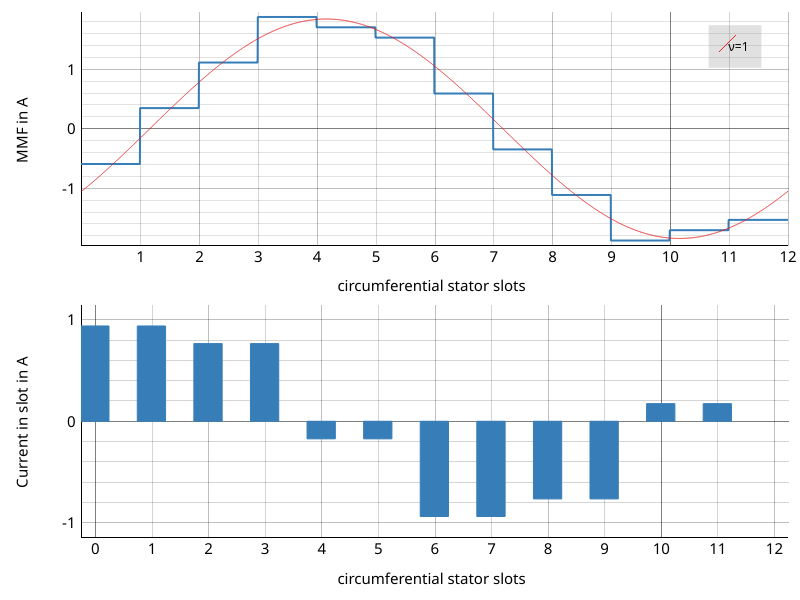Plot of the current linkage in the slots and the resulting Magnetomotove force with phaseangle = 20°.

## File IO¶

After creating a winding we can save it as a *.wdg file This file can be used with the GUI for example. swat-em uses the “json” format for the *.wdg files.

```>>> wdg = datamodel()
>>> wdg.genwdg(Q = 12, P = 2, m = 3, layers = 1)
>>> wdg.save_to_file('myfile.wdg')
```

We can also load an existing winding from file:

```>>> wdg2 = datamodel()
```

Proof, that the data of the two objects is equal:

```>>> print('same data?:', wdg.machinedata == wdg2.machinedata)
same data?: True
>>> print('same results?:', wdg.results == wdg2.results)
same results?: True
```

### Export to Excel file¶

The data of an existing winding can exported to an Excel file (*.xlsx). Attention: The old *.xls format is not supported!

```>>> wdg.export_xlsx('export.xlsx')
```

### Text report¶

A summary of the winding can be exported as a text report:

```>>> wdg.export_text_report('report.txt')
```

### HTML report¶

Similar to the text report we can create a html report. This also includes the graphics.

```>>> wdg.export_html_report('report.html')
```